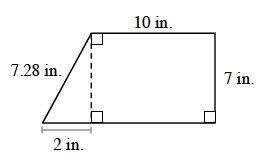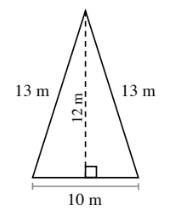### Home > CC1MN > Chapter 11 > Lesson 11.2.2 > Problem11-47

11-47.

Find the area and perimeter of each shape. Show your work.

1.1.• Perimeter: What are the unlabeled lengths?

Area: What is the base and the height of the triangle?

Use the formulas below to find the area of each section.
$b=\text{base}$
$h = \text{height}$

$\text{Area of a Triangle} = \frac{1}{2}bh$

$\text{Area of a Rectangle} = (b)(h)$

Then, add the area of the triangle with the area of the rectangle.

Perimeter: $36.28$ in
Area: $77$ in2

• Perimeter: Add the lengths of all of the sides around the outside of the figure.

Area: What is the base and height of the triangle?
Using the area formula for a triangle (from part (a)), find the area.